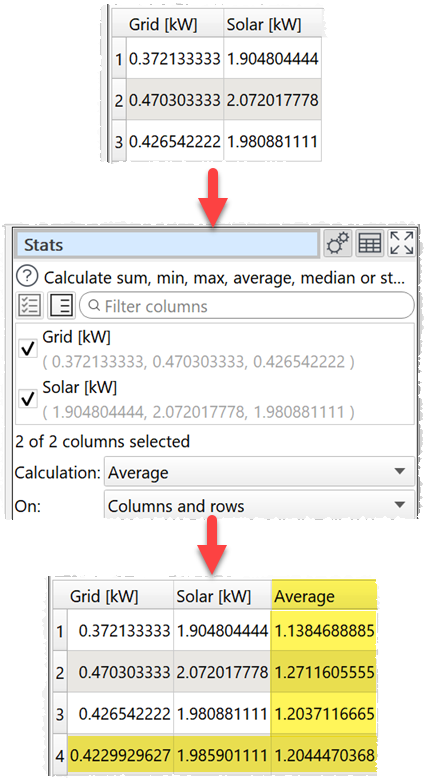﻿ Reference > Transforms > Stats

# Stats

## Description

Calculates statistics by column and/or row in one or more selected columns.

## Example

Calculate column and row averages:One.

## Options

Check the column(s) you wish to calculate stats for.

Set Calculation to the statistic you want to calculate:

oSum show the sum of the values.

oMinimum shows the smallest value.

oMaximum shows the largest value.

oAverage shows the arithmetic mean of the values.

oMedian shows the median of numeric values in the column.

oMode shows the mode of numeric values in the column.

oStandard deviation is the sample standard deviation (equivalent to Excel function stddev.s).

oVariance is the square of the sample standard deviation.

oQ1 is the first quartile (calculated using Method 1 here).

oQ3 is the third quartile  (calculated using Method 1 here).

oIQR is the Inter Quartile Range.

oSkew is the alternative Pearson Mode Skewness  (3 * (Mean – Median) / Standard Deviation ).

Set On depending on whether you wish to calculate the statistics for columns, rows or both.

oIf On is set to Columns an extra row with the results is added to the bottom.

oIf On is set to Rows an extra column with the results is added to the right.

oIf On is set to Columns and rows  an extra row with the results is added to the bottom and extra column with the results is added to the right. The bottom right cell contains the calculation across all values.

## Notes

Non-numerical and empty values are ignored.

Mode may not work as expected with non-integer values, due to precision issues. You can fix this by using a Num Format transform first.

Use Num Format to change the precision of the results.

Use Scale to convert the results to percentages.

If you want to compute the sum, minimum, maximum or average for each distinct value in 1 or more columns you can use Unique.